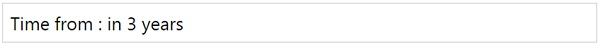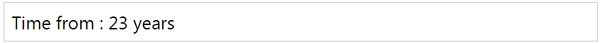# MomentJS - Time to now

This method tells the length of time from the date given to now.

## Syntax

```moment().toNow();
moment().toNow(Boolean);
```

## Example

```var a = moment([2015, 3, 15]).toNow();
```

## OutputIf you want to remove the prefix, use true with toNow() as shown below −

## Example

```var a = moment([1995, 3, 15]).toNow(true);
```

## Outputmomentjs_formatting_date_and_time.htm